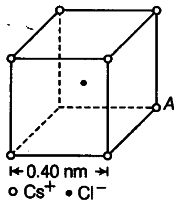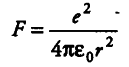# Figure represents a crystal unit of caesium chloride

Figure represents a crystal unit of caesium chloride, CsCl. The caesium atoms, represented by open circles are situated at the corners of a cube of side 0.40 nm, whereas a Cl atom is situated at the centre of the cube. The Cs atoms are deficient in one electron while the Cl atom carries an excess electron.
(i) What is the net electric field on the Cl atom due to eight Cs atoms?
(ii) Suppose that the Cs atom at the comer A is missing. What is the net force now on the Cl atom due to seven remaining Cs atoms?(i)From the given figure, we can analyse that the chlorine atom is at the centre of the cube, i.e. at equal distance from all the eight corners of cube where caesium atoms are placed. Thus, due to symmetry, the force due to all Cs atoms, on Cl atom will cancel out.
Hence , E = F / q’
where, F=0,
Therefore,E=0
(ii)Thus, net force on Cl atom at A would be given bywhere, r = distance between Cl ion and Cs ion. Applying Pythagoras theorem, we get